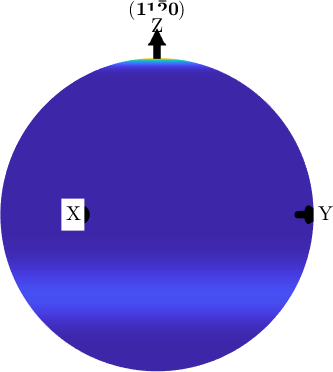Spherical Projections edit page

MTEX supports four types of spherical projection which are available for all spherical plot, e.g. polefigure plots, inverse polefigure plots or ODF plots. These are the equal area projection (Schmidt projection), the equal distance projection, the stereographic projection (equal angle projection), the three-dimensional projection and the flat projection.

In order to demonstrate the different projections we start by defining a model ODF.

## Alignment of the Hemispheres

Partial Spherical Plots

If an ODF has triclinic specimen symmetry its pole figures differs in general on the upper hemisphere and the lower hemisphere. By default MTEX plots, in this case, both hemispheres. The upper on the left-hand side and the lower on the right-hand side. TODO: this is currently missing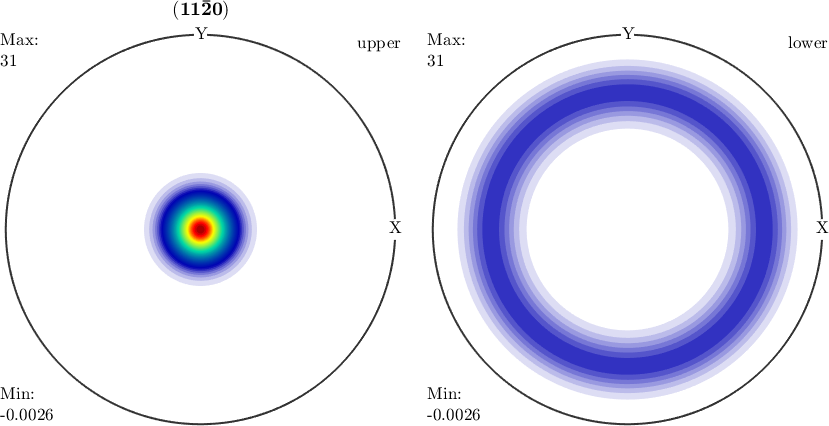MTEX allows also to plot only the upper or the lower hemisphere by passing the options upper or lower.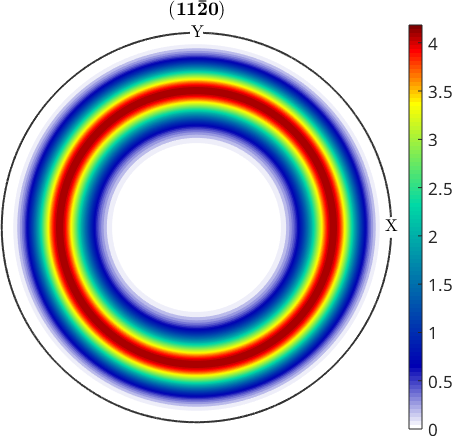Due to Friedel's law measured pole figures are a superposition of the upper and the lower hemisphere (since antipodal directions are associated). In order to plot pole figures as a superposition of the upper and lower hemisphere one has to enforce antipodal symmetry. This is done by the option antipodal.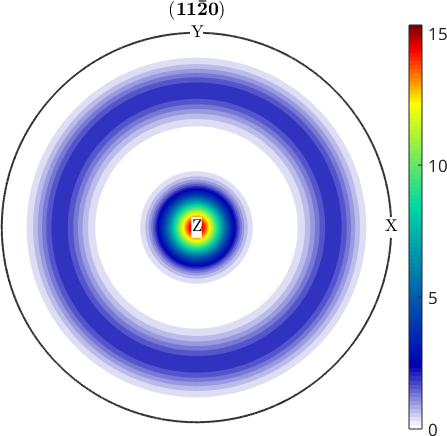## Alignment of the Coordinate Axes

Rotate and Flip Plots

Sometimes it is more convenient to have the coordinate system rotated or flipped in some way. For this reason, all plot commands in MTEX allows for the options rotate, flipud and fliplr. A more direct way for changing the orientation of the plot is to specify the direction of the x-axis by the commands plotx2east, plotx2north, plotx2west, plotx2south.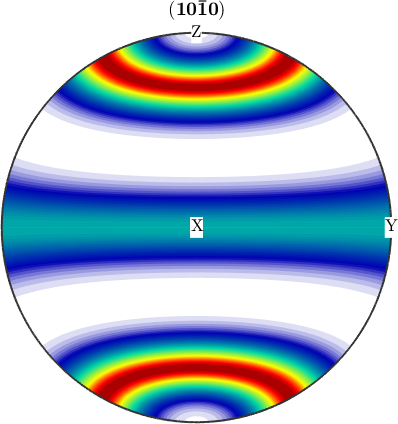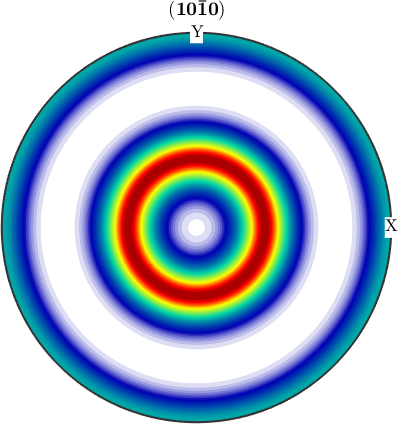## Equal Area Projection (Schmidt Projection)

Equal area projection is defined by the characteristic that it preserves the spherical area. Since pole figures are defined as relative frequency by area equal area projection is the default projection in MTEX. In can be set explicitly by the flags earea or schmidt.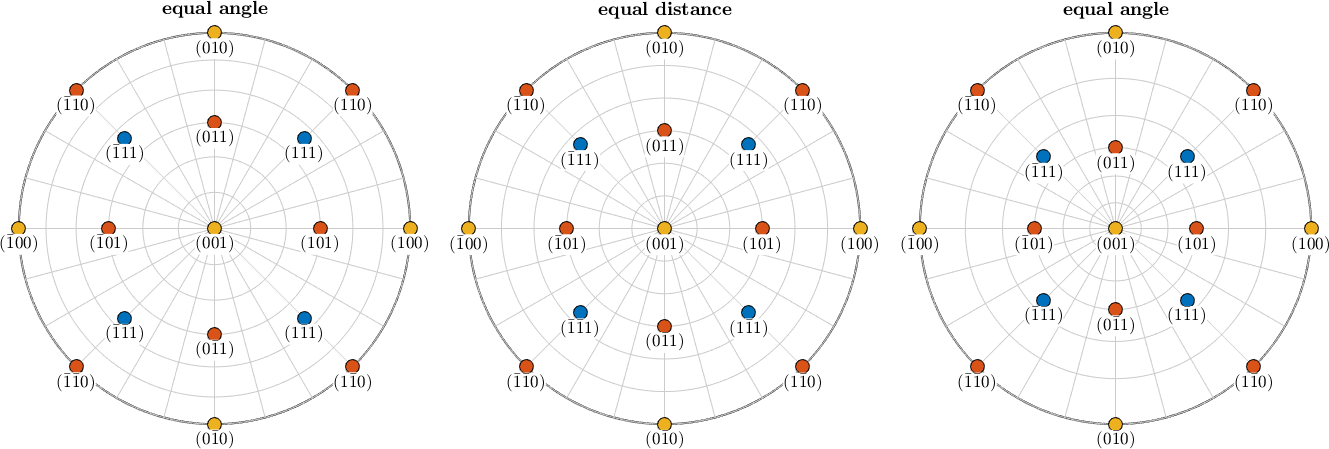## Equal Distance Projection

The equal distance projection differs from the equal area projection by the characteristic that it preserves the distances of points to the origin. Hence it might be a more intuitive projection if you look at crystal directions.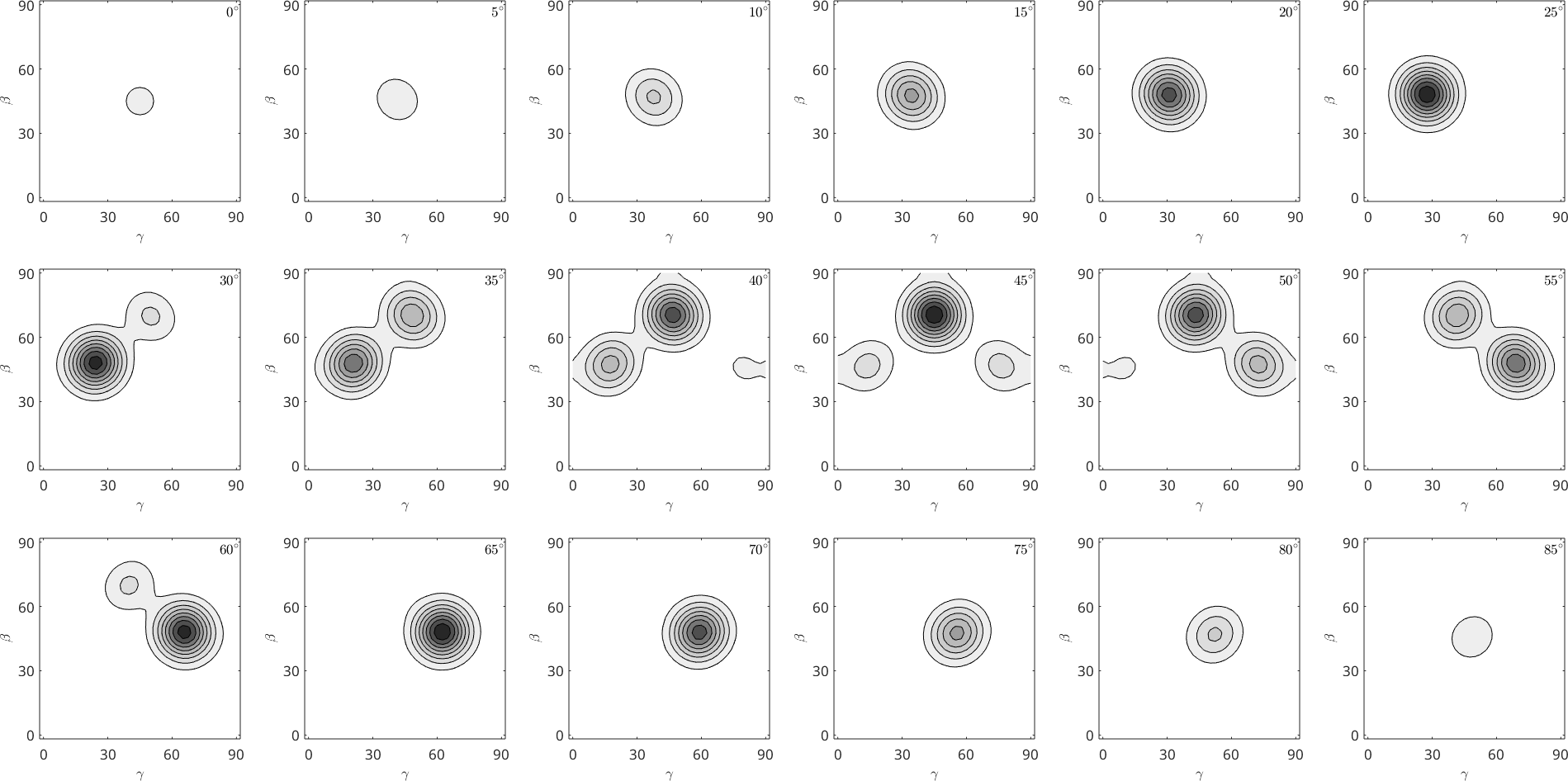## Stereographic Projection (Equal Angle Projection)

Another famous spherical projection is the stereographic projection which preserves the angle between arbitrary great circles. It can be chosen by setting the option stereo or eangle.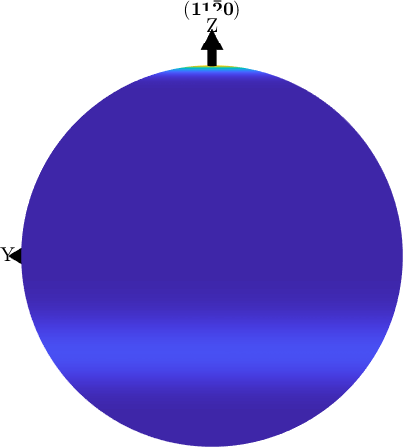## Plain Projection

Plain means that the polar angles theta / rho are plotted in a simple rectangular plot. This projection is often chosen for ODF plots, e.g.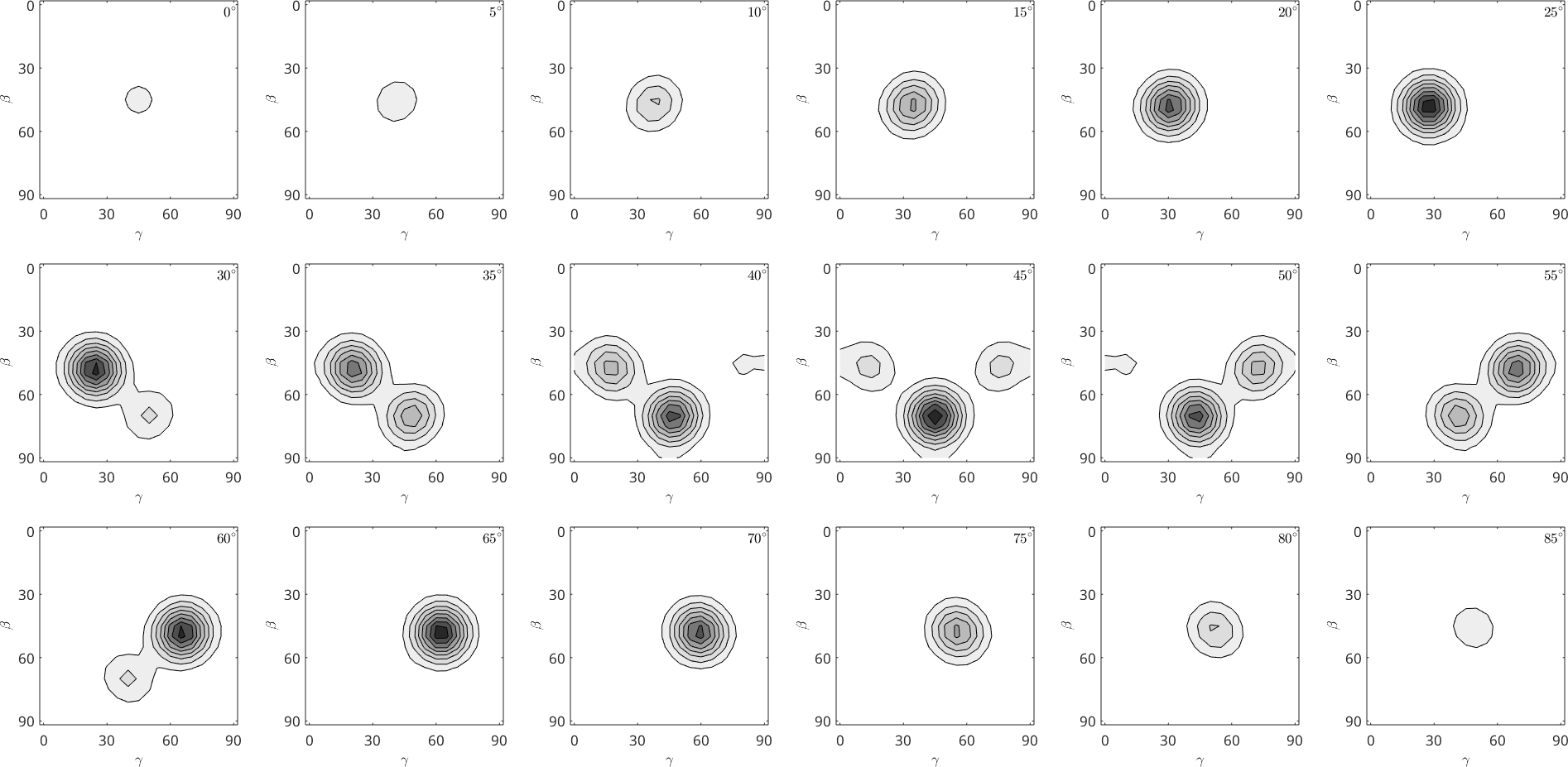## Three-dimensional Plots

MTEX also offers a three-dimensional plot of pole figures which even might be rotated freely in space